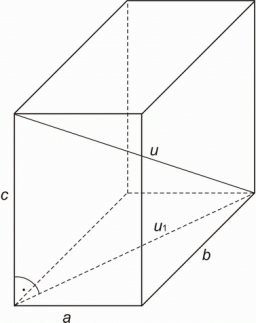# Space diagonal angles

Calculate the angle between the body diagonal and the side edge c of the block with dimensions: a = 28cm, b = 45cm and c = 73cm. Then, find the angle between the body diagonal and the plane of the base ABCD.

C =  35.9807 °
A =  54.0193 °

### Step-by-step explanation:Did you find an error or inaccuracy? Feel free to write us. Thank you!Tips to related online calculators
Pythagorean theorem is the base for the right triangle calculator.
Most natural application of trigonometry and trigonometric functions is a calculation of the triangles. Common and less common calculations of different types of triangles offers our triangle calculator. Word trigonometry comes from Greek and literally means triangle calculation.

#### You need to know the following knowledge to solve this word math problem:

We encourage you to watch this tutorial video on this math problem:

## Related math problems and questions:

• CuboidCuboid ABCDEFGH with 10 cm height has a base edge length 6 cm and 8 cm. Determine angle between body diagonal and the base plane (round to degrees).
• Four sided prismCalculate the volume and surface area of a regular quadrangular prism whose height is 28.6cm and the body diagonal forms a 50-degree angle with the base plane.
• Body diagonalCalculate the length of the body diagonal of a block with dimensions: a = 20 cm, b = 30 cm, c = 15 cm.
• RectangleCalculate the length of the side GN and diagonal QN of rectangle QGNH when given: |HN| = 25 cm and angle ∠ QGH = 28 degrees.
• Diagonals of a prismThe base of the square prism is a rectangle with dimensions of 3 dm and 4 dm. The height of the prism is 1 m. Find out the angle between the body diagonal with the diagonal of the base.
• Body diagonalCuboid with base 7cm x 3,9cm and body diagonal 9cm long. Find the height of the cuboid and the length of the diagonal of the base,The regular quadrilateral pyramid has a base edge a = 1.56 dm and a height h = 2.05 dm. Calculate: a) the deviation angle of the sidewall plane from the base plane b) deviation angle of the side edge from the plane of the base
• Right angleIn a right triangle ABC with a right angle at the apex C, we know the side length AB = 24 cm and the angle at the vertex B = 71°. Calculate the length of the legs of the triangle.
• Angle of diagonalThe angle between the body diagonal of a regular quadrilateral and its base is 60°. The edge of the base has a length of 10cm. Calculate the body volume.Calculate the volume (V) and the surface (S) of a regular quadrilateral prism whose height is 28.6 cm and the deviation of the body diagonal from the base plane is 50°.
• DiagonalDetermine the dimensions of the cuboid, if diagonal long 53 dm has an angle with one edge 42° and with another edge 64°.
• Base diagonalIn a regular 4-sided pyramid, the side edge forms an angle of 55° with the base's diagonal. The length of the side edge is eight meters. Calculate the surface area and volume of the pyramid.
• Cube - anglesCalculate the angle between the wall diagonal and cube base. Calculate the angle between the cube body diagonal and cube base.
• Pyramid 8Calculate the volume and the surface area of a regular quadrangular pyramid with the base side 9 cm and side wall with the base has an angle 75°.
• Body diagonalCalculate the volume of a cuboid whose body diagonal u is equal to 6.1 cm. Rectangular base has dimensions of 3.2 cm and 2.4 cm
• Height of the cuboidCuboid with a rectangular base, measuring 3 cm and 4 cm diagonal has a body 13 centimeters long. What is the height of the cuboid?
• BoxCalculate the angle between box base 9 x 14 and body diagonal length 18.